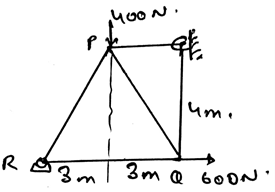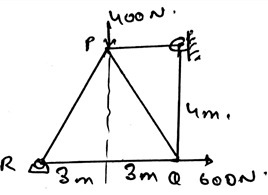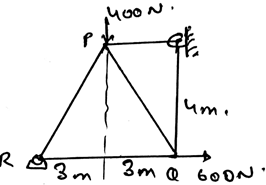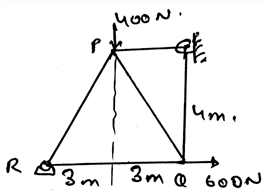Courses

# Test: Method Of Joints

## 15 Questions MCQ Test Engineering Mechanics | Test: Method Of Joints

Description
This mock test of Test: Method Of Joints for Mechanical Engineering helps you for every Mechanical Engineering entrance exam. This contains 15 Multiple Choice Questions for Mechanical Engineering Test: Method Of Joints (mcq) to study with solutions a complete question bank. The solved questions answers in this Test: Method Of Joints quiz give you a good mix of easy questions and tough questions. Mechanical Engineering students definitely take this Test: Method Of Joints exercise for a better result in the exam. You can find other Test: Method Of Joints extra questions, long questions & short questions for Mechanical Engineering on EduRev as well by searching above.
QUESTION: 1

### If the whole truss is in equilibrium then all the joints which are connected to that truss is in equilibrium. This is known as:

Solution:

If the whole truss is in equilibrium then all the joints which are connected to that truss is in equilibrium. This is known as method of joints. This is one of the methods to solve for the forces which are acting along the trusses.

QUESTION: 2

### Find the force in the member vertical at the right, joined by the hinge.Solution:

The direction of the unknown is not known to us. To know the direction of the unknown force we take assumption of it. That is we assume that the particular direction might be the direction of the force and then we do the calculations accordingly. And then we apply the equilibrium equations to the joints.

QUESTION: 3

### The free body diagram of which part of the section of the truss is made to make use of method of joints?

Solution:

The free body diagram of joints part of the section of the truss is made to make use of method of joints. Various equations of equilibrium are made to do the calculations. The net force acting on the vertical direction is made zero. Also horizontal direction forces are made to be zero.

QUESTION: 4

For applying the method of joint at joints the forces need to coplanar.

Solution:

The forces are coplanar that’s why they are there in the calculations in the method of joints. The forces are resolved in 2D. This makes the condition of the equilibrium fulfilled and hence the method of joints uses the forces in the same plane for its calculation.

QUESTION: 5

For applying the method of joint at joints the forces need to be concurrent.

Solution:

The forces are concurrent that’s why they are there in the calculations in the method of joints. The forces are resolved in 2D. This makes the condition of the equilibrium fulfilled by cancelling of various force members and hence the method of joints uses the concurrent forces for its calculation.

QUESTION: 6

Find the force in the member RQ.Solution:

The direction of the unknown is not known to us. To know the direction of the unknown force we take assumption of it. That is we assume that the particular direction might be the direction of the force and then we do the calculations accordingly. And then we apply the equilibrium equations to the joints.

QUESTION: 7

We use the method of joint to find the forces acting over the joints. In this we start from the joint having at least one known force and at the most two unknown forces.

Solution:

This makes the calculations easy. Also for the solving of any equation one requires equal number of variables as that of equal number of equation. So to do the calculations easily the above mentioned conditions are followed.

QUESTION: 8

We use the method of joint to find the net force acting over the entire structure.

Solution:

We use this method for the simplification of the forces in the sections of the structure. Not the entire structure. This means that the statement given is wrong. It should be the joints not the whole structure.

QUESTION: 9

Which of the following is correct?

Solution:

The direction of the unknown is not known to us. To know the direction of the unknown force we take assumption of it. That is we assume that the particular direction might be the direction of the force and then we do the calculations accordingly.

QUESTION: 10

The magnitude of the unknown force can’t be known. But the direction can be founded out.

Solution:

The first part is wrong. We can determine the magnitude of the unknown force. It can be done by using the equilibrium equations. That equating the forces zero, of the three different axis. Thus making the components of the unknown founded out.

QUESTION: 11

Find the force in the member PQ.Solution:

The direction of the unknown is not known to us. To know the direction of the unknown force we take assumption of it. That is we assume that the particular direction might be the direction of the force and then we do the calculations accordingly. And then we apply the equilibrium equations to the joints.

QUESTION: 12

What is after taking the assumption of the direction of the force, the direction comes opposite?

Solution:

The direction is comes opposite, means that the assumption was wrong. But that doesn’t means that the question can’t be solved further. One needs to change the sign of the direction and the question is solved. Thus the calculations of the force direction.

QUESTION: 13

The resultant of three equal vectors having mutual angles being 120 degree and being originated from a single point is zero.

Solution:

As one can imagine three vectors coming out of a single point and are having a 120 degree angle with their adjacent ones, the resultant would be zero.

QUESTION: 14

The magnitude of the resultant of the two vectors is always:

Solution:

Yes, the magnitude of the resultant of the two vectors always depends on the angle between them. It might be greater or smaller than one of the vector’s length. For perfectly saying, it does depends upon the angle between them.

QUESTION: 15

Find the force in the member PR.Solution:

The direction of the unknown is not known to us. To know the direction of the unknown force we take assumption of it. That is we assume that the particular direction might be the direction of the force and then we do the calculations accordingly. And then we apply the equilibrium equations to the joints.